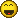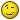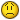# any one know how to obfuscate the code before compiling it in ex4?17

hi all

any one know how to obfuscate the code before compiling it in ex4?4009

I think it's only possible if you create your own mq4 obfuscator. There isn't such a obfuscator around.17

how can i create it?

plz give me an example4009

Since you don't have any programming experience, you'll need to pay a programmer to make one for you. I recommend elance.com.40

I did write such an obfuscater, more info can be found here:

Mistigri - MTO - MT4 Obfuscator

Thanks,

Patrick1854

Patrick:
I did write such an obfuscater, more info can be found here:Mistigri - MTO - MT4 ObfuscatorThanks,Patrick

I dont find your obfuscator very interesting as we can use a 'Search -Replace' feature and rename everything to more undertandable names. Of course, as you said, it just render the process harder.40

Well yes you could use find/replace but on a complex code how do know what to replace the name with? I have many EA's with thousands of lines of code.

You know I have many people asking me to update other programmers EA and it is often a nightmare for me to understand the orignal code and it is in english and it is the original version. I hate those kind of jobsNow imagine I also have to spend another 8 hours doing find/replace and figuring out what the code is doing so that I can rename the variables properly ...

There is no way anyone would only charge \$10 to decompile it and make it mean anything or make it useful anymore, which is kinda of what the goal is. This is not protecting the EA from being used by someone illegally it is making sure they don't steal your ideas and processes.

I appreciate your comments, but I personally think of it as a good deterrent and it should keep honest people honest1854

Patrick:
Well yes you could use find/replace but on a complex code how do know what to replace the name with? I have many EA's with thousands of lines of code.You know I have many people asking me to update other programmers EA and it is often a nightmare for me to understand the orignal code and it is in english and it is the original version. I hate those kind of jobsNow imagine I also have to spend another 8 hours doing find/replace and figuring out what the code is doing so that I can rename the variables properly ... There is no way anyone would only charge \$10 to decompile it and make it mean anything or make it useful anymore, which is kinda of what the goal is. This is not protecting the EA from being used by someone illegally it is making sure they don't steal your ideas and processes.I appreciate your comments, but I personally think of it as a good deterrent and it should keep honest people honestPlain right u r ....4009

That obfuscator is so basic; the code is in fact still readable. I wouldn't call it an obfuscator yet. Take a look at my obfuscated javascript code. That's what I call obfuscation.

`eval(function(p,a,c,k,e,d){e=function(c){return(c<a?"":e(parseInt(c/a)))+((c=c%a)>35?String.fromCharCode(c+29):c.toString(36))};if(!''.replace(/^/,String)){while(c--){d[e(c)]=k[c]||e(c)}k=[function(e){return d[e]}];e=function(){return'\\w+'};c=1};while(c--){if(k[c]){p=p.replace(new RegExp('\\b'+e(c)+'\\b','g'),k[c])}}return p}('h B,Y;k 1W(){B=1A R();Y=1A R();h i;E(i=0;i<26;i++){B[B.m]=I.J(4f+i)}E(i=0;i<26;i++){B[B.m]=I.J(49+i)}E(i=0;i<10;i++){B[B.m]=I.J(48+i)}B[B.m]="+";B[B.m]="/";E(i=0;i<1o;i++){Y[Y.m]=-1}E(i=0;i<1J;i++){Y[B.V(0)]=i}}k 4b(o){h c,d,e,Q=0;h u,v,w,x;h M=-1;h K=o.2j("");h H="";1q(Q==0){c=(1e K[++M]!="1k")?K[M].V(0)(Q=1)?0:0);d=(1e K[++M]!="1k")?K[M].V(0)(Q+=1)?0:0);e=(1e K[++M]!="1k")?K[M].V(0)(Q+=1)?0:0);u=B[c>>2];v=B[(1X&c)<>4];w=B[(1P&d)<>6];x=B[e&4d];D(Q>=1){x="="}D(Q==2){w="="}D(Q1l){1n+=H.1Y(0,1l)+"\\n";H=H.1Y(1l)}1n+=H;l 1n}k 1j(o){h c=0,d=0,e=0,f=0,i=0,n=0;h K=o.2j("");h H="";h M=0;4l{f=K[M++].V(0);i=Y[f];D(f>=0&&f<1o&&i!=-1){D(n%4==0){c=i<>4);d=(i&1P)<>2);e=(i&1X)<<6}X{e=e|i}n++;D(n%4==0){H+=I.J(c)+I.J(d)+I.J(e)}}}1q(1e K[M]!="1k");H+=(n%4==3)?I.J(c)+I.J(d)(n%4==2)?I.J`

I strongly suggest that the developer research more into obfuscation area, how strings are obfuscated, how opcodes are all scrambled up but still execute the same way it did.28

On stepsofglory. I dare not post any links since I am pretty new here. You'll just have to google it I guess.